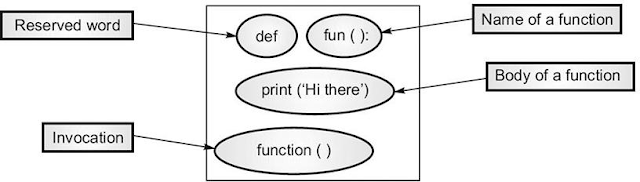# Python How to Create Function

In Python, you can define and call the function. Once done, you can call it later from the other program. I have shared the structure of it. And, I have added the three rules of it; useful for interviews.

## Structure of FunctionStructure of Function

### Rules to Create a Function

1. A function definition is executable.
2. The body will have the code to perform the task you wish.
3. You can assign default parameter values.

### 1. Key Elements.

You will find here three key elements of the Python function. Here you can read the parameter vs argument differences.

Name of a function. It can be any valid identifier. It should be meaningful, and it should convey the work it will do.

Parameter. These should be separated with command and should be in the parentheses following the name of the function. The parameters are input to function. It can have any parameters.

Body of the function. The function body can contain code that implements the task to be performed by it.

### 2. Sample Function.

Python Example.

def product(num1, num2):
# defined function name of product with two input parameters.
# And this 'def' ends with ':' colon.

prod= num1*num2
# multiplying two numbers num1 and num2 and assign to prod

print('The product of the numbers is \t:'+str(prod))
# prints product of two numbers

num1=int(input('Enter the first number\t:'))
num2=int(input('Enter the second number\t:'))
return prod
# returns prod value to the calling program.

### 3. How to call a function.

myProduct = product(num1, num2)
# supply two arguments to the product function.

print(myProduct)
#The product function prints the product of two numbers.

References